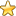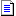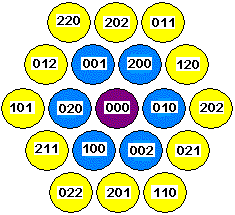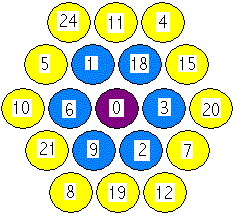You are here: HomeThe Esoteric Section# Base 3 and the Cube

The 27 three digit strings can be divided into groups depending apon the number of zeros in the string.

1 string contains three zeros.

6 strings contain two zeros.

12 strings contain one zero.

8 strings contain no zeros.

 Base 10 Base 3 0 000 1 001 2 002 3 010 4 011 5 012 6 020 7 021 8 022 9 100 10 101 11 102 12 110 13 111 14 112 15 120 16 121 17 122 18 200 19 201 20 202 21 210 22 211 23 212 24 220 25 221 26 222

These groups of 1, 6, 12 and 8 correspond exactly with the features of the cube:

The 8 strings which contain no zeros correspond with the 8 vertices of the cube.

The 12 strings which contain one zero correspond with the 12 edges of the cube.

The 6 strings which contain two zeros correspond with the 6 faces of the cube.

The 1 string which contains three zeros corresponds with the center of the cube.There is a progression from the outer-most features containing no zeros to the center which contains 3 zeros thus:

The corners contain no zeros.

Moving inwards, the next feature of the cube,
the edges, correspond with strings containing one zero.
Nearer the center again,
the faces, correspond with strings containing two zeros.
The string corresponding with
the center corresponds with the string composed entirely of zeros.

Here is the same cube translated into base 10.Note that it adds to 39 along every edge and the 3 diagonal directions through the center. The sum of the faces=39. If each two digit base 10 number is added together to form a single number a pattern emerges.

The 19 base 3 figures that contain at least 1 zero form a magic hexagon which adds up to 39 in every direction.You are here: HomeThe Esoteric SectionSearch on Amazon:
esoteric or...?